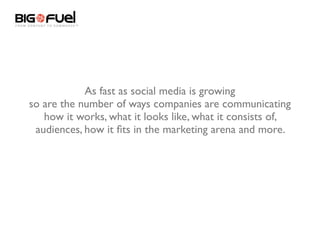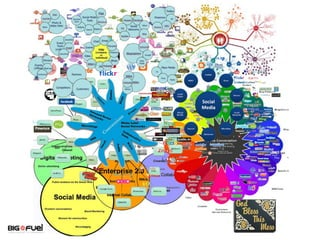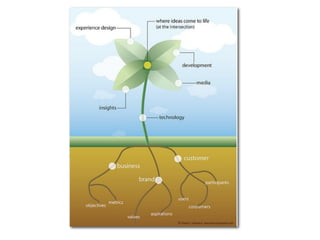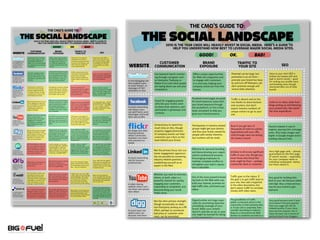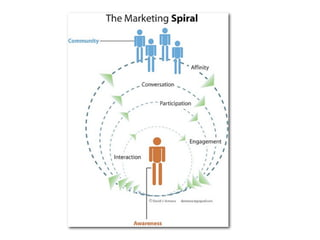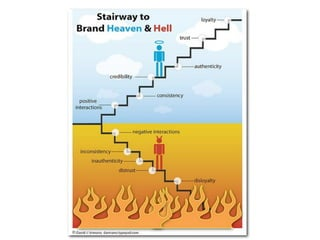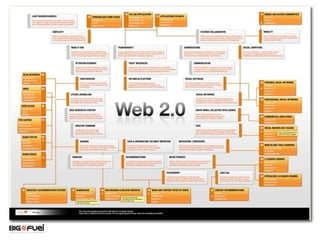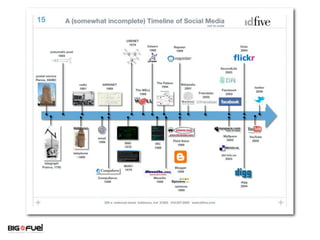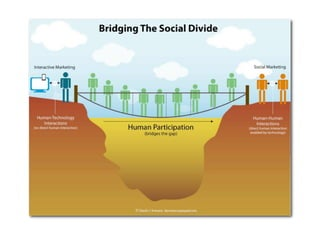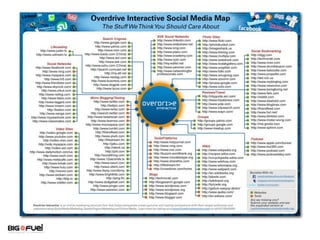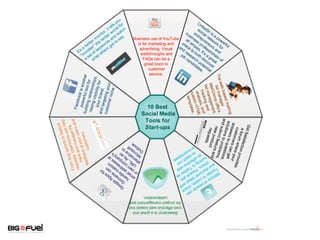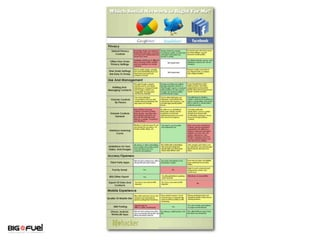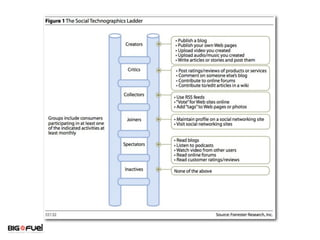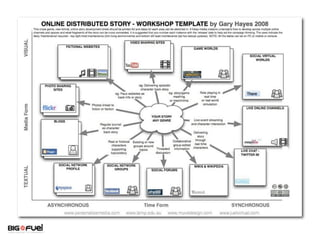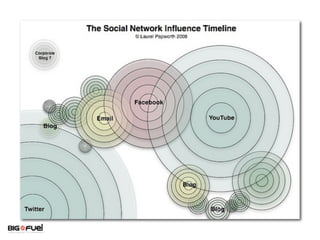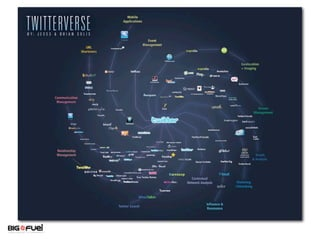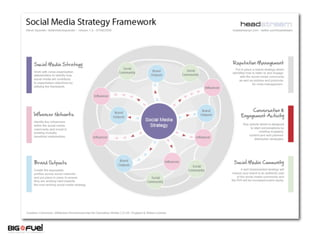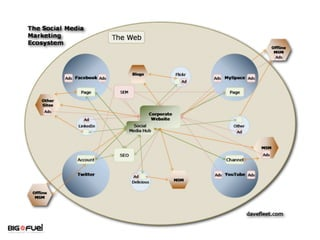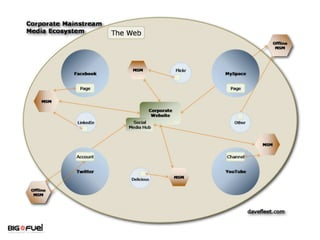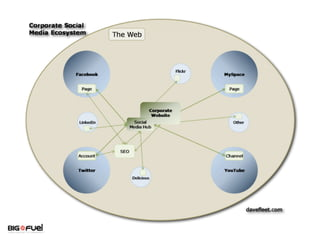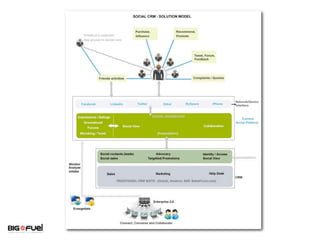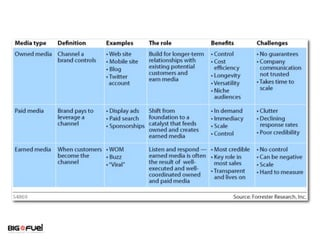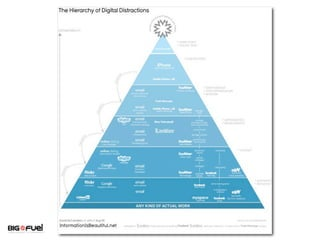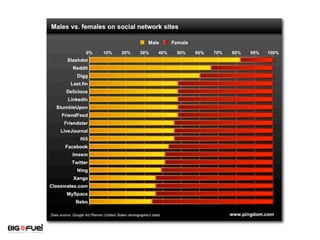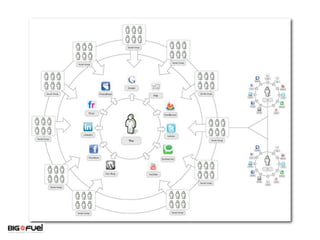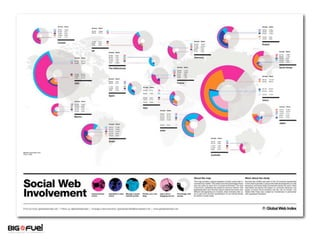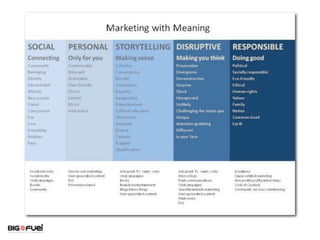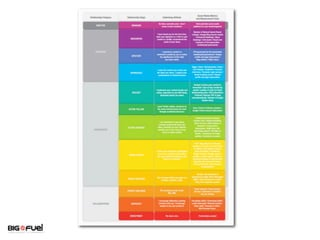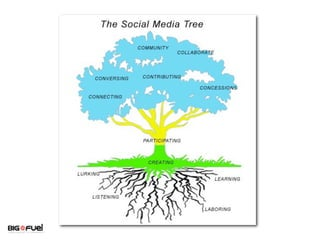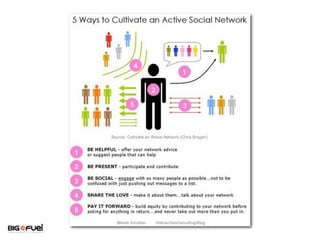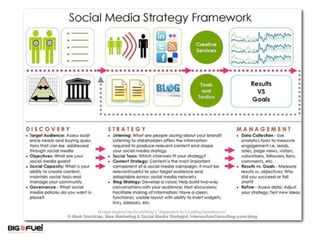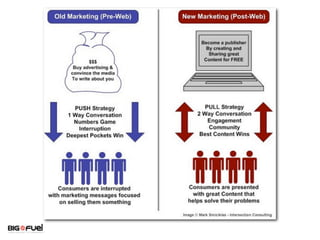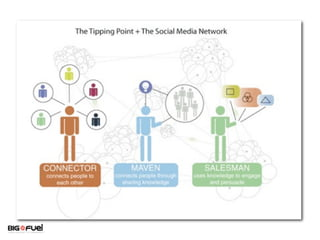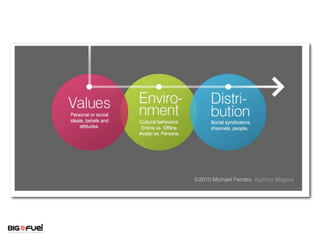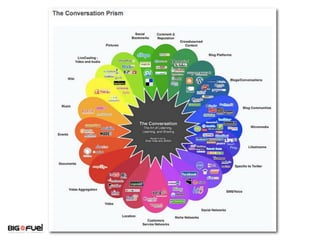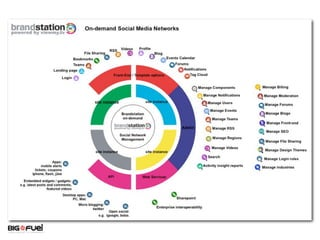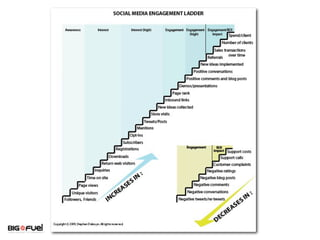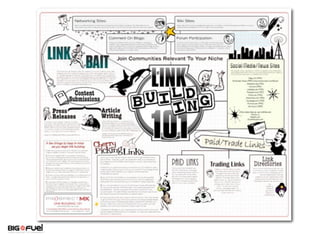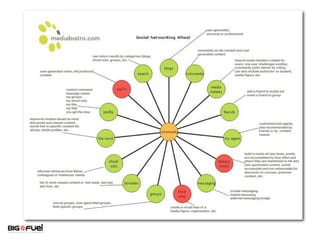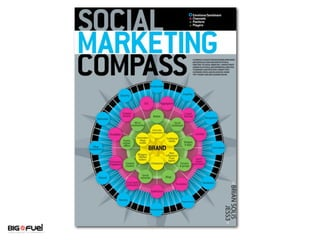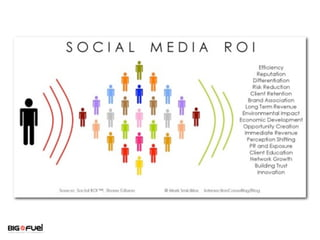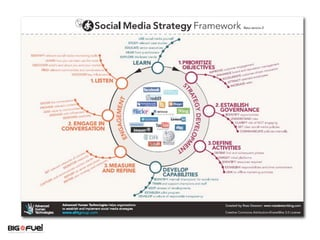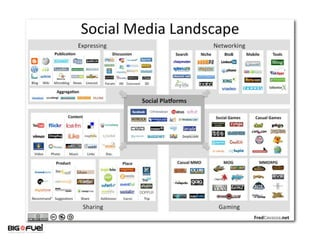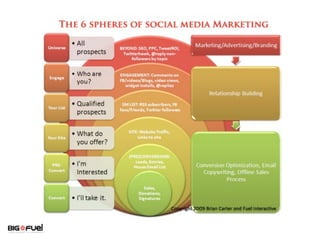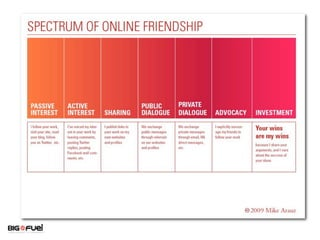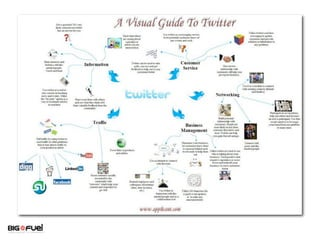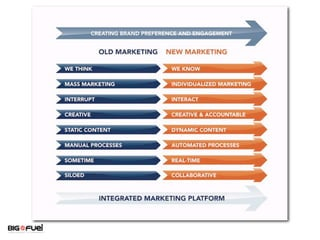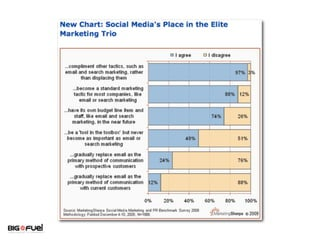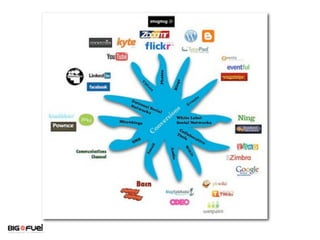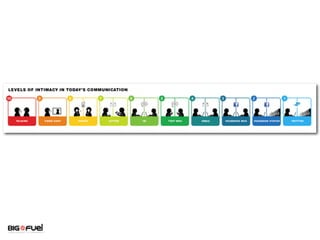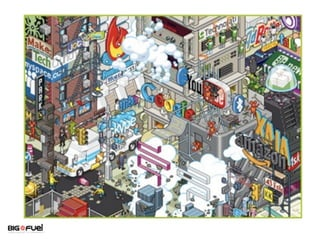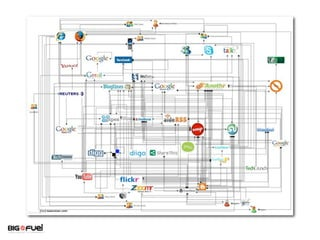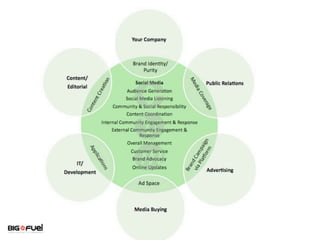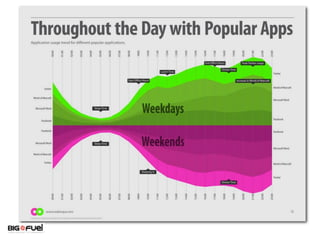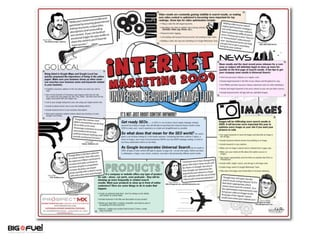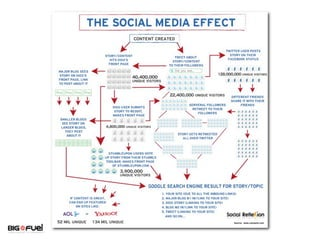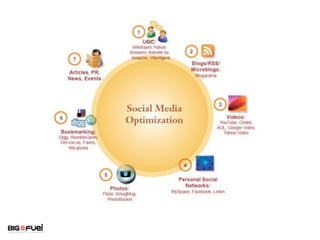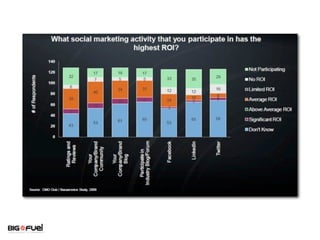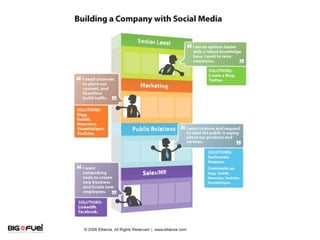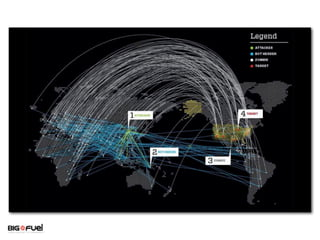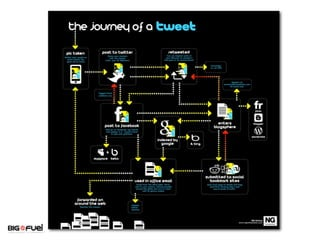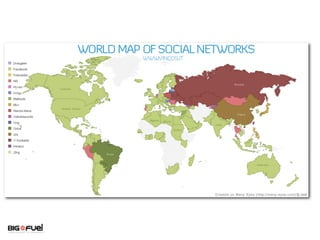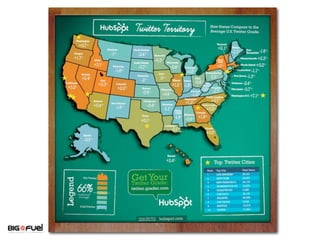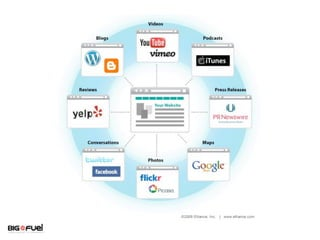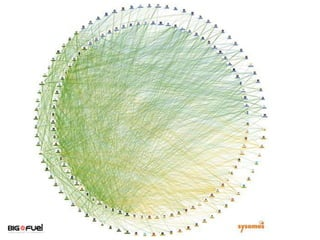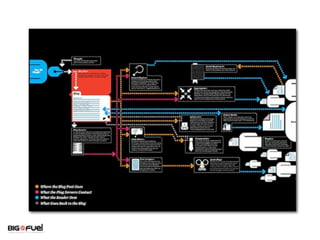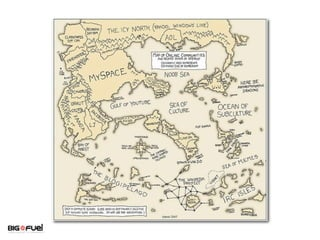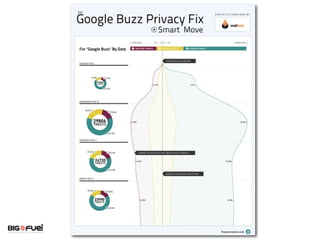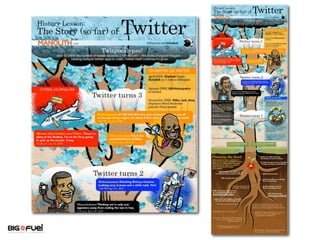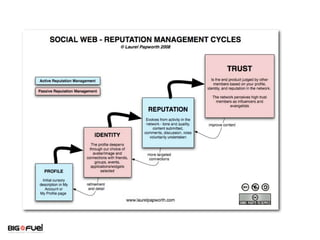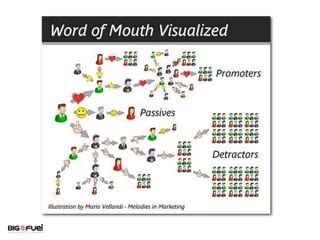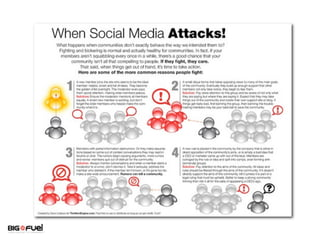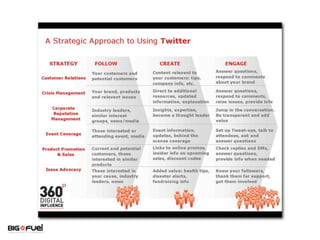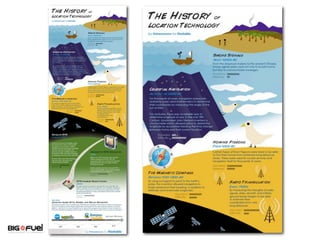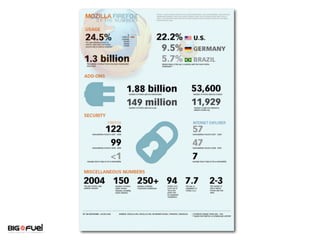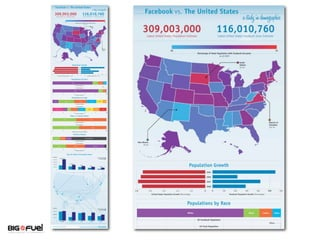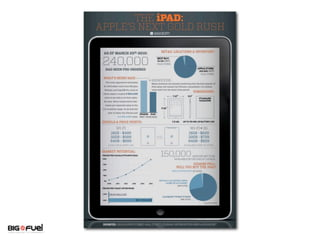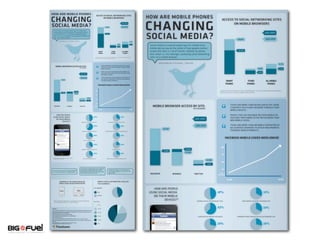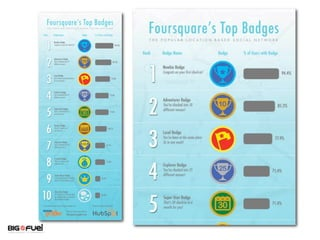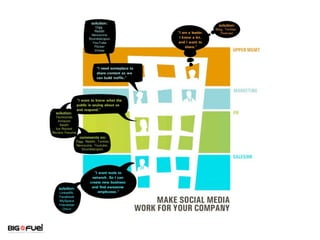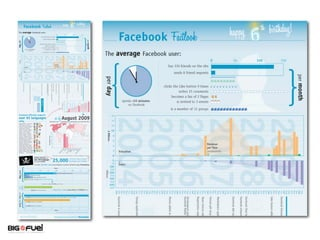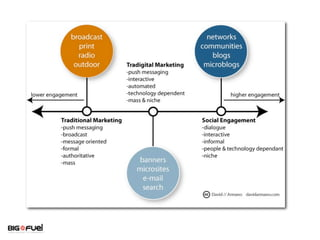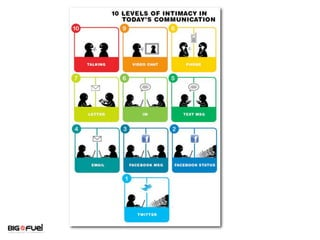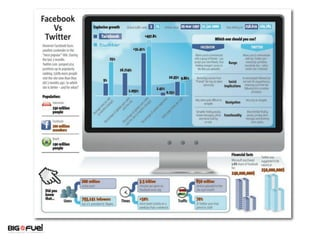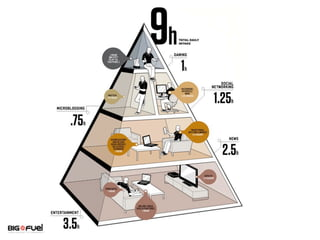1 of 86

### Social Media Charts

1. This is where an appropriate headline will go. 85 awesome, useful and beautiful social media infographics
2. This is where an appropriate headline will go. As fast as social media is growing so are the number of ways companies are communicating how it works, what it looks like, what it consists of, audiences, how it ﬁts in the marketing arena and more.
3. This is where an appropriate headline will go.
4. This is where an appropriate headline will go.
6. This is where an appropriate headline will go.
7. This is where an appropriate headline will go.
8. This is where an appropriate headline will go.
9. This is where an appropriate headline will go.
10. This is where an appropriate headline will go.
11. This is where an appropriate headline will go.
12. This is where an appropriate headline will go.
13. This is where an appropriate headline will go.
14. This is where an appropriate headline will go.
15. This is where an appropriate headline will go.
16. This is where an appropriate headline will go.
17. This is where an appropriate headline will go.
18. This is where an appropriate headline will go.
19. This is where an appropriate headline will go.
20. This is where an appropriate headline will go.
21. This is where an appropriate headline will go.
22. This is where an appropriate headline will go.
23. This is where an appropriate headline will go.
24. This is where an appropriate headline will go.
25. This is where an appropriate headline will go.
26. This is where an appropriate headline will go.
27. This is where an appropriate headline will go.
28. This is where an appropriate headline will go.
29. This is where an appropriate headline will go.
30. This is where an appropriate headline will go.
31. This is where an appropriate headline will go.
32. This is where an appropriate headline will go.
33. This is where an appropriate headline will go.
34. This is where an appropriate headline will go.
35. This is where an appropriate headline will go.
36. This is where an appropriate headline will go.
37. This is where an appropriate headline will go.
38. This is where an appropriate headline will go.
39. This is where an appropriate headline will go.
40. This is where an appropriate headline will go.
41. This is where an appropriate headline will go.
42. This is where an appropriate headline will go.
43. This is where an appropriate headline will go.
44. This is where an appropriate headline will go.
45. This is where an appropriate headline will go.
46. This is where an appropriate headline will go.
47. This is where an appropriate headline will go.
48. This is where an appropriate headline will go.
49. This is where an appropriate headline will go.
50. This is where an appropriate headline will go.
51. This is where an appropriate headline will go.
52. This is where an appropriate headline will go.
53. This is where an appropriate headline will go.
54. This is where an appropriate headline will go.
55. This is where an appropriate headline will go.
56. This is where an appropriate headline will go.
57. This is where an appropriate headline will go.
58. This is where an appropriate headline will go.
59. This is where an appropriate headline will go.
60. This is where an appropriate headline will go.
61. This is where an appropriate headline will go.
62. This is where an appropriate headline will go.
63. This is where an appropriate headline will go.
64. This is where an appropriate headline will go.
65. This is where an appropriate headline will go.
66. This is where an appropriate headline will go.
67. This is where an appropriate headline will go.
68. This is where an appropriate headline will go.
69. This is where an appropriate headline will go.
70. This is where an appropriate headline will go.
71. This is where an appropriate headline will go.
72. This is where an appropriate headline will go.
73. This is where an appropriate headline will go.
74. This is where an appropriate headline will go.
75. This is where an appropriate headline will go.
76. This is where an appropriate headline will go.
77. This is where an appropriate headline will go.
78. This is where an appropriate headline will go.
79. This is where an appropriate headline will go.
80. This is where an appropriate headline will go.
81. This is where an appropriate headline will go.
82. This is where an appropriate headline will go.
83. This is where an appropriate headline will go.
84. This is where an appropriate headline will go.
85. This is where an appropriate headline will go.
86. This is where an appropriate headline will go.